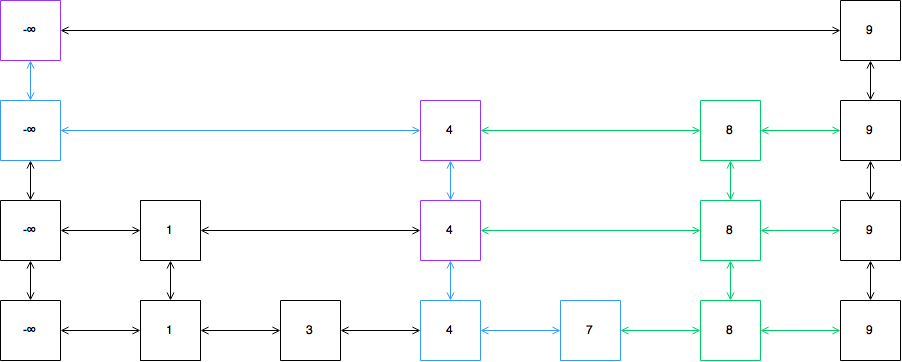# Implementing Skip Lists

Skip lists are interesting data structures that rely on a random element in its design. The resulting data structure allows O(log(n)) time complexity for search, insert, and delete operations with high probability. Here is a walkthrough of a skip list implementation, also available on my Github repo.

## Background/Concept

The general concept of a skip list is an improved version of a linked list. We start out with a simple sorted linked list as shown below. A search algorithm for a simple linked list has worst-case O(n) time complexity, since each node needs to be examined to search for the target value.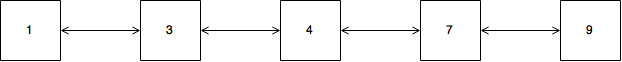An improvement upon a linked list would be “express lines”. Essentially, another list that contains only a subset of the elements of the main list. Using the “express line”, I can traverse the list faster. I call them “express lines” because they function very similarly to express lines for trains. If my goal was to travel to stop 7, I wouldn’t take the “local” line that goes through 1, 3, and 4 before finally reaching 7. I should take the express line from 1 to 4, then 4 to 7. Or maybe 1 to 9, then 9 to 7. Fewer stops = faster “search”.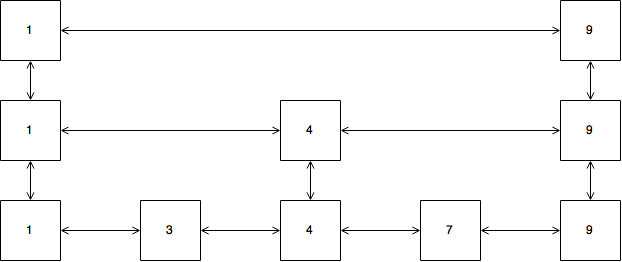This is the basic concept with skip lists. With this new data structure, I can create a search algorithm by starting at the upper left node (which is called the head):

1. Walk right unless the node to the right is greater than the target or does not exist
2. If right node is too big or does not exist, walk down
3. Repeat until value is found, or if step 1 and 2 are not possible, then the value does not exist

Using this algorithm, searching for 7 would go through these steps (highlighted in blue in the diagram):

• Start at 
• Moving right  is greater than  -> move down to 
• Moving right  is less than  -> move right to 
• Moving right  is greater than  -> move down to 
• Moving right is equal to  -> move right to 
• Done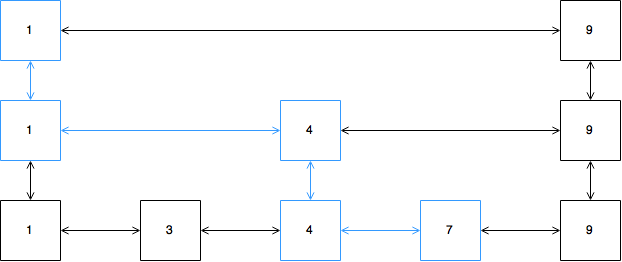The best search algorithm for this data structure is no longer O(n) time complexity. In fact, if I have n nodes on the bottom list, and roughly half as many evenly spaced nodes on the second list, and so on, the time complexity for the search algorithm is average O(log(n)).

Note that I said evenly spaced nodes for each of the “express” lists. This is trivial to set up if the nodes are known in advance, but as with any useful data structure, the availability of this information should not be relied upon. So how do I determine which nodes to “promote” into the express lists? It’s impossible to predict what numbers will be inserted into my skip list, so there’s no knowing if my choices will result in an evenly spaced list. So the best we can do is flip a (fair) coin. Heads - promote up one level. Repeat until I get tails.

The MIT lecture on skip lists is a great resource for going deeper into the math, but the bottom line is that the design I have proposed will ensure, with high probability, that search/insert/delete operations can be done in O(log(n)) time complexity.

## Implementation

### Nodes

Now we need the nodes that will be used for the skip list. These are similar to the nodes of a doubly linked list, except instead of just `previous` and `next` links, they also need `above` and `below` links. I’ll go with `up`, `down`, `left`, `right` for sake of easily aligning them with the steps of the algorithm.

``````var Node = function(value) {
this.value = value;
};

Node.prototype.insertAfter = function(node) {
// inserts `this` after the node argument
this.right =  node.right;
node.right && (node.right.left = this); // only assign if node.right exists
node.right =  this;
this.left  =  node;
};

Node.prototype.stackOnTop = function(node) {
// places `this` on top of node argument
this.up    =  node.up;
node.up    && (node.up.down = this);
node.up    =  this;
this.down  =  node;
};

Node.prototype.remove = function() {
this.down  && (this.down.up = this.up);
this.left  && (this.left.right = this.right);
this.right && (this.right.left = this.left);
this.up    && (this.up.down = this.up);
};
``````

The `Node` class has several methods for inserting and removing nodes. The `insertAfter` method does the following to a list: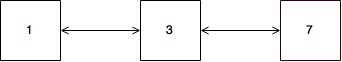``````var five = new Node(5);
five.insertAfter(three);
``````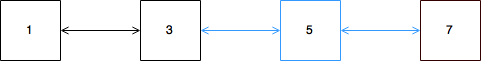### Skip List

As mentioned earlier, the skip list needs a head, which is the node that all search, insert, and delete algorithms will start form. The head needs to be the upper left most node and must be at the highest level. The only logical value for this is `-Infinity`.

``````var Skiplist = function() {
};
``````

Here’s an example skip list. Let’s use this to implement our `search` method.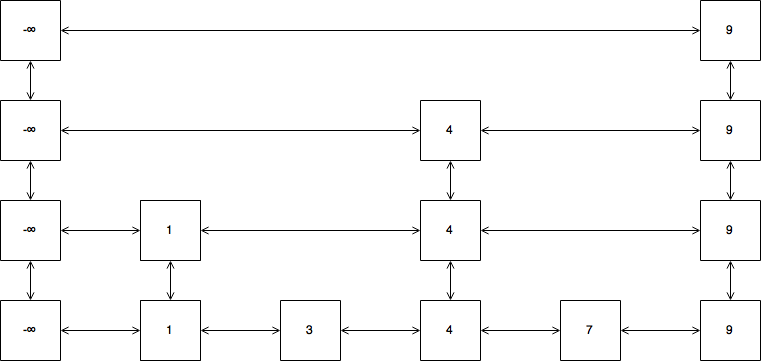Here is the recursive search algorithm. This is the exact same algorithm that I described above. I used an inner recursive function that I am invoking immediately with the head node as a starting point. `at` refers to whatever node I am currently examining.

``````Skiplist.prototype.search = function(value) {
return (function search(at, value) {
// if value is found, return true
if( at.value === value ) return true;

// if right is smaller, go right
if( at.right && at.right.value <= value ) {
return search(at.right, value);
}

// otherwise, go down if possible
if( at.down ) {
return search(at.down, value);
}

// cannot go right or down, the value is not in the skip list
return false;
};
``````

For the example skip list, here is the traversal for `search(8)`. Once the 7 node is reached, the right node is greater than the target, and there are no more nodes below, so it is concluded that 8 is not in the skip list - `search` returns `false`.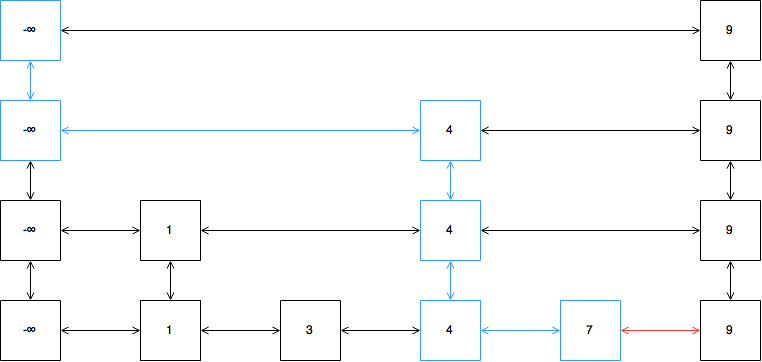### Remove/Delete

The delete operation goes through many of the same steps as search. After all, the node must be found before it can be deleted. The only difference is that when the node is found, instead of simply returning `true`, the deletion needs to be performed. I will show the delete operation later, but you can see how the remaining logic is identical. Note that I `call`ed the inner `remove` function with a binding to `this`, which has to do with the deletion step.

``````Skiplist.prototype.remove = function(value) {
return function remove(at, value) {
// value found -> perform delete
if( at.value === value ) {
// do delete
}

// if right is smaller, go right
if( at.right && at.right.value <= value ) {
return remove.call(this, at.right, value);
}

// otherwise, go down if possible
if( at.down ) {
return remove.call(this, at.down, value);
}

return false;
};
``````

Due to the structure of skip lists, the node at the highest level for the value I’m searching for is reached first. So `at` is the highest level node matching the value I am attempting to delete. I need to traverse down and remove every node that matches the value to complete the deletion. At the same time, for any node I remove, if there are no other nodes on the level except for the head (such as the highest level 9 in the diagram above), I also need to redirect the `head` pointer to the next level down.

``````while( at ) {
at.remove();
// if nothing else is on this level and not on level 1
// set head to next level down
}
at = at.down;
}
// remove complete
return true;
``````

Since I am reassigning the `head` pointer. I need a reference to the instance of the skip list, hence the `call` binding.

The complete `remove` method looks like this:

``````Skiplist.prototype.remove = function(value) {
return function remove(at, value) {
// value found -> perform delete
if( at.value === value ) {
while( at ) {
at.remove();
// if nothing else is on this level // and not on level 1
// set head to next level down
}
at = at.down;
}
// remove complete
return true;
}

// if right is smaller, go right
if( at.right && at.right.value <= value ) {
return remove.call(this, at.right, value);
}

// otherwise, go down if possible
if( at.down ) {
return remove.call(this, at.down, value);
}

return false;
};
``````

### Insert

The first thing we need is a coin (let’s assume `Math.random()` is fair):

``````var coinFlip = function() {
return Math.random() < 0.5;
};
``````

Again, the `insert` method is very similar to the `search` algorithm as well. The difference being that I need to insert a value once I reach a node where there are no more nodes to the right or the right node is greater than the value I am trying to insert, and I cannot traverse down to a lower level. That is, where I would return `false` in the `search` method, I need to instead perform an insert.

One thing to note and verify for yourself is that unlike `remove`, once I am at this location, I am always on the base level.

Finally, if the value is found during this search process, then the value already exists in the skip list and I should return `false`.

``````Skiplist.prototype.insert = function(value) {
var drops = []; // *
return function insert(at, value) {
// value already exists, insertion fail
if( at.value === value ) return false;

// if right is smaller, go right
if( at.right && at.right.value <= value ) {
return insert.call(this, at.right, value);
}

// otherwise, go down if possible
if( at.down ) {
drops.push(at); // *
return insert.call(this, at.down, value);
}

// do insert
};
``````

One thing you may have noticed is the new `drops` array. This is what it is for: once I arrive at the location to insert my new node, I want to insert it after `at`. The next step of the algorithm is to flip a coin, and promote the value accordingly. It is easy enough to link it vertically the node I just inserted, but how should I insert them to the levels above? Enter the `drops`. This is the same diagram as above for the search algorithm, except I’ve highlighted in purple each node where I move down.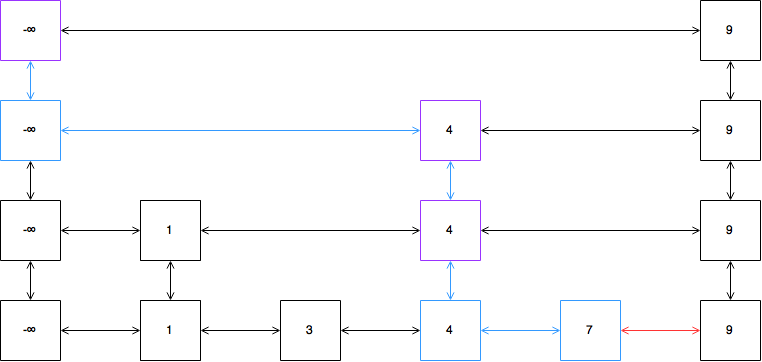These are the nodes that I add to the `drops` array, and are in fact the nodes after which I would insert the promoted nodes. If by chance, I am promoting above the highest current level, then I need to create a new node of `-Infinity` and reassign the `head` pointer. And for the same reason as the `delete` method, I need to use `call` and bind it to the skip list instance.

``````var base = new Node(value);
base.insertAfter(at);

while( coinFlip() ) {
var promote = new Node(value);
promote.stackOnTop(base);
base = promote;

var drop = drops.pop();
if( drop ) {
promote.insertAfter(drop);
} else {

}
}

// insert complete
return true;
``````

The full `insert` method looks like this:

``````Skiplist.prototype.insert = function(value) {
var drops = [];
return function insert(at, value) {
// value already exists, insertion fail
if( at.value === value ) return false;

// if right is smaller, go right
if( at.right && at.right.value <= value ) {
return insert.call(this, at.right, value);
}

// otherwise, go down if possible
if( at.down ) {
drops.push(at);
return insert.call(this, at.down, value);
}

// if can't go down, insert
var base = new Node(value);
base.insertAfter(at);

while( coinFlip() ) {
var promote = new Node(value);
promote.stackOnTop(base);
base = promote;

var drop = drops.pop();
if( drop ) {
promote.insertAfter(drop);
} else {

}
}

// insert complete
return true;
As an example, invoking `mySkipList.insert(8)` might result in a skip list looking like this, where the nodes and links in green are the result of the insert operation: